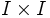# Difference between revisions of "Two sides lemma"

This term is nonstandard and is being used locally within the wiki. For its use outside the wiki, please define the term when using it.

## Statement

The inclusion of two adjacent sides in the unit square is equivalent to the inclusion of one side in the unit square, viz., in the inclusion of$I$ in$I \times I$.

An explicit isotopy can also be written down, which may be more convenient in some situations.

## Applications

• The inclusion of a point in the unit interval is a cofibration. To prove this, we note that this boils down to proving that two adjacent sides of the square are a retract of the unit square, which, by the two sides lemma, is equivalent to requiring that$I$ is a retract of$I \times I$ which is clearly true.

The retraction from$I \times I$ to two sides can also be written down explicitly; this is more useful at times.